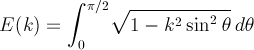Computes the complete elliptic integral of the second kind.

Namespace:  Meta.Numerics.Functions
Assembly:  Meta.Numerics (in Meta.Numerics.dll) Version: 4.1.4Syntax
```public static double EllipticE(
double k
)```

#### Parameters

k
Type: SystemDouble
The elliptic modulus, which must lie between zero and one.

#### Return Value

Type: Double
The value of the Legendre integral E(k).Exceptions
ExceptionCondition
ArgumentOutOfRangeExceptionk lies outside [0, 1].Remarks

E(k) is defined as the complete elliptic integral:It appears in the Legendre reduction of integrals of rational funtions.

The perimeter of an ellipse with major axis a and eccentricity e is 4 a E(e).

Be aware that some authors use the the parameter m = k2 instead of the modulus k.See Also